### Question 8. Let the period of revolution of a planet at a distance R from a star be T. Prove that if it was at a distance of 2R from the star, its period of revolution will be √8 T.

Question 8.

Let the period of revolution of a planet at a distance R from a star be T. Prove that if it was at a distance of 2R from the star, its period of revolution will be √8 T.

By Kepler’s Third law of planetary motion;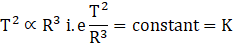Where ‘r’ is the distance of planet from the star; ‘T’ is the period of revolution.

Let T1 be the time period when planet was at a distance of ‘2R’ from the star.

By Kepler’s third law;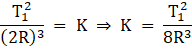Since K is constant and its value is same therefore;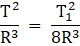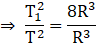⇒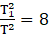⇒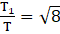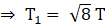Hence Period of revolution will be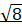times the period of revolution when planet was at distance ‘R’ from the star.